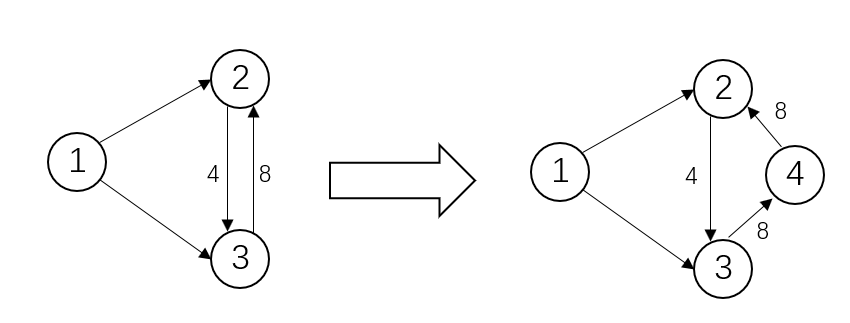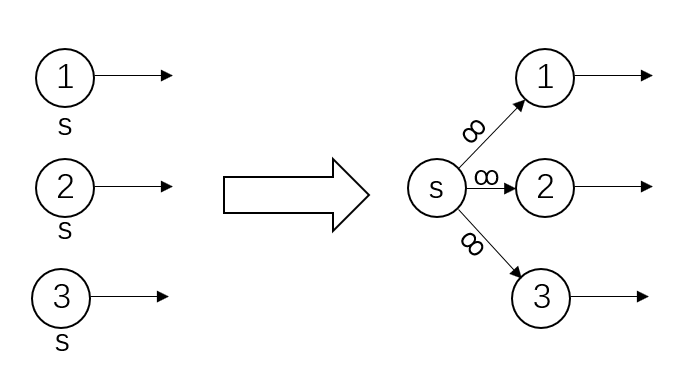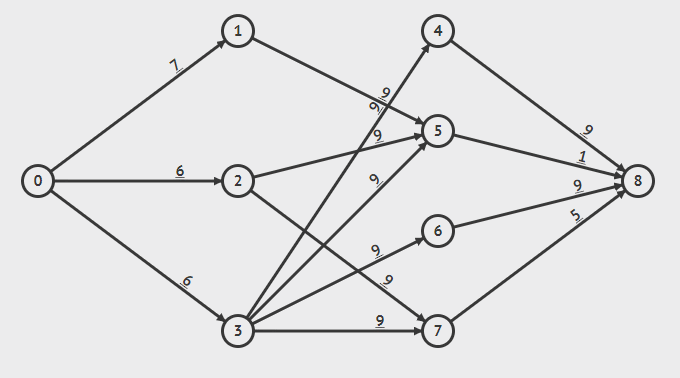# 最大流问题

## Maxflow Problem

ZingLix June 16, 2018

## 一、定义

### 流网络

• 每条边都有一个 非负 的容量值 $$c(u,v)$$
• 如果存在一条边（u, v），那么 不存在 反向的边（v, u）
• 图中有两个特殊节点，源结点（source）汇点(sink)

### 流

• 容量限制：$$\forall u, v \in V, 0 \le f(u,v) \le c(u,v)$$，即每条边传递量要小于边的容量
• 流量守恒：$$\forall u \in V - \{s,t\}, \sum_{v \in V} f(v,u) = \sum_{v \in V} f(u,v)$$，即除源结点和汇点，流入等于流出

$\vert f \vert = \sum_{v \in V} f(s,v) - \sum_{v \in V} f(v, s)$

## 二、特殊情况处理

### 反向边### 多源结点 & 多汇点## 三、相关概念

### 残存网络

$c_f (u, v) = \begin{cases} c(u, v) - f(u, v), & (u, v) \in E \\ f(v, u), & (v, u) \in E \\ 0 & 其他 \end{cases}$### 增广路径

$c_f (p) = min \{c_f(u,v)\} , (u,v) \in p$

### 流网络的切割$f(S,T) = \sum_{u \in S} \sum_{v \in T} f(u,v) - \sum_{u \in S} \sum_{v \in T} f(v,u)$

$c(S,T) = \sum_{u \in S} \sum_{v \in T} c(u,v)$

• 设 $$(S,T)$$ 为流网络的任意切割，那么净流量 $$f(S,T) = \vert f\vert$$
• 流网络 $$G$$ 中任意流 $$f$$ 的值不超过 $$G$$ 的任意切割的容量
• 如下几个条件等价：
• $$f$$ 是 $$G$$ 的一个最大流
• 残存网络中不含任何增广路径
• $$\vert f \vert = c(S,T)$$，其中 $$(S,T)$$为某个切割

## 四、Ford-Fulkerson 算法

1
2
3
4
5
6
7
8
9
10
11
12
13
14
ford_fulkerson(G,s,t){
for(edge(u,v) in G.E){
(u,v).f = 0
}
while(残存网络中存在增广路径 p){
for(edge(u,v) in p){
if((u,v) 存在){
(u,v).f = (u,v).f + c_f(p)  //c_f(p) 为 p 的残存容量
}else{
(v,u).f = (v,u).f - c_f(p)
}
}
}
}Gif 根据visugo.net 制作